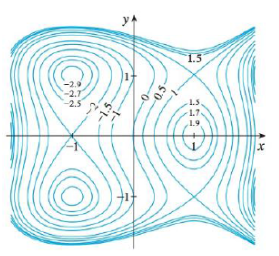Chapter 14.7, Problem 4E

Chapter
Section
Textbook Problem

Use the level curves in the figure to predict the location of the critical points of f and whether f has a saddle point or a local maximum or minimum at each critical point. Explain your reasoning. Then use the Second Derivatives Test to confirm your predictions.4. f(x, y) = 3x − x3 − 2y2 +3 y4To determine

To predict: The location of the critical points of f and whether f has a local maximum, local minimum and saddle point at each critical point using the level curves in the figure. Use second derivative test to confirm the predictions.

Explanation

Result used:

Second Derivative Test:

“Suppose the second partial derivatives of f are continuous on a disk with center (a,b) , and suppose that fx(a,b)=0 and fy(a,b)=0 (that is (a,b) is a critical point of f).

Let D=D(a,b)=fxx(a,b)fyy(a,b)[fxy(a,b)]2

(a) If D>0 and fxx(a,b)>0 , then f(a,b) is a local minimum.

(b) If D>0 and fxx(a,b)<0 , then f(a,b) is a local maximum.

(c) If D<0 , then f(a,b) is not a local maximum or minimum and it is called a saddle point”.

Calculation:

From the given figure it is clear that the points (1,1),(1,1) are surrounded by an oval shape.

Also, it can be identified that the value of f is increasing around all the points of (1,±1) .

Thus, the local minimum occurs at (1,±1) or near the point (1,±1) . Therefore, the critical point is (1,±1) .

The function is decreasing at the point (1,0) and hence it is local maximum

Moreover observe that near the points (1,0),(1,1),(1,1) , the values of f is increasing in some directions and decreasing in some other direction. So, there exists the saddle points at (1,0),(1,1),(1,1) .

Verification:

The given function is, f(x,y)=3xx32y2+y4 .

Take the partial derivative in the given function with respect to x and obtain fx .

fx=x(3xx32y2+y4)=x(3x)x(x3)x(2y2)+x(y4)=3(1)3x20+0=33x2

Thus, fx=33x2 . (1)

Take the partial derivative in the given function with respect to y and obtain fy .

fy=y(3xx32y2+y4)=y(3x)y(x3)y(2y2)+y(y4)=002(2y)+4y3=4y+4y3

Thus, fy=4y+4y3 . (2)

Set the above partial derivatives to 0 and find the values of x and y.

From the equation (1),

33x2=03x2=3x2=1x=±1

From the equation (2),

4y+4y3=04y(y21)=0y=0,y2=1y=0,y=±1

Thus, the critical points are, (1,0),(1,1),(1,1),(1,0),(1,1) and (1,1) .

Take the partial derivative of the equation (1) with respect to x and obtain fxx .

2fx2=x(33x2)=x(3)x(3x2)=03(2x)=6x

Hence, 2fx2=6x .

Take the partial derivative of the equation (2) with respect to y and obtain fyy

2fy2=y(4y+4y3)=y(4y)+y(4y3)=4(1)+4(3y2)=4+12y2

Hence, 2fy2=4+12y2 .

Take the partial derivative of the equation (1) with respect to y and obtain fxy .

2fxy=y(33x2)=y(3)y(3x2)=00=0

Hence, 2fxy=0

Still sussing out bartleby?

Check out a sample textbook solution.

See a sample solution

The Solution to Your Study Problems

Bartleby provides explanations to thousands of textbook problems written by our experts, many with advanced degrees!

Get Started

Find more solutions based on key concepts